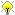[Documentation] [TitleIndex] [WordIndex]Please ask about problems and questions regarding this tutorial on answers.ros.org. Don't forget to include in your question the link to this page, the versions of your OS & ROS, and also add appropriate tags.

# Polynomials

Description: Working with polynomials of the n-th degree.

Keywords: ecl polynomials

Tutorial Level: INTERMEDIATE

Next Tutorial: Splines

## The Polynomial Class

Polynomials are a template class where the template argument represents the degree of the polynomial. The class stores only the co-efficients for the polynomial and calculates the function and its derivatives on the fly from these co-efficients. Coefficient storage is based on the array class in ecl_containers, so comma initialisation can be used to configure the polynomial appropriately.

The following instantiates an uninitialised polynomial. Use comma initialisation or blueprints to initialise.

```   1 Polynomial<5> p;
```

## Typedefs

Rather than specifying the degree of the polynomial with a template argument, a few more convenient typedefs are available:

```   1 typedef Polynomial<1> LinearFunction;
3 typedef Polynomial<3> CubicPolynomial;
4 typedef Polynomial<5> QuinticPolynomial;
```

## Initialisation

### Comma Initialisation

```   1 Polynomial<5> p;
2 // Comma Initialisation
3 p.coefficients() = 1,2,3,4,5,6;
4 cout << p << endl; // 1.00 + 2.00x + 3.00x^2 + 4.00x^3 + 5.00x^4 + 6.00x^5
5
```

### BluePrints

There are also some blueprints (quick construction and assignment) for generating polynomials that interpolate between two end points. These can be accessed via static methods that are inherited by the polynomial's class. For example:

```   1 LinearFunction linear = LinearFunction::Interpolation(0.0,0.0,1.0,2.0);                         // x_i, y_i, x_f, y_f
2 LinearFunction linear = LinearFunction::PointSlopeForm(1.0,2.0,2.0);                            // x_f, y_f, slope
3 CubicPolynomial cubic;
4 cubic = CubicPolynomial::DerivativeInterpolation(2.0,0.0,0.0,3.0,1.0,0.0);                      // x_i, y_i, y'_i, x_f, y_f, y'_f
5 cubic = CubicPolynomial::SecondDerivativeInterpolation(2.0,0.0,0.0,3.0,1.0,0.0);                // x_i, y_i, y''_i, x_f, y_f, y''_f
6 QuinticPolynomial quintic = QuinticPolynomial::Interpolation(0.0,0.0,0.0,0.0,1.0,2.0,1.0,0.0);  // x_i, y_i, y'_i, y''_i, x_f, y_f, y'_f, y''_f
7
```

### Shifting

There is also a method for shifting the polynomial on the horizontal axis. A negative offset will shift the polynomial to the left, while a positive offset will shift the polynomial to the right.

```   1 Polynomial<2> quadratic; // x^2
2 quadratic.shift(2);      // x^2 -> (x-2)^2 (graph shifted right by 2)
3
```

Later, if there is a need a vertical shift will be added.

## Derivatives

```   1 Polynomial<5> p;
2 // Comma Initialisation
3 p.coefficients() = 1,2,3,4,5,6;
4 cout << p << endl; // 1.00 + 2.00x + 3.00x^2 + 4.00x^3 + 5.00x^4 + 6.00x^5
5
6 // Derivatives
7 Polynomial<4> pdot = p.derivative();  // the derivative function
8 double pdot_five = p.derivative(5.0); // value of the derivative
9 Polynomial<2> pddot = p.dderivative();
10 double pddot_five = p.dderivative(5.0); // value of the derivative
11
```

## Polynomial Functions

There also exist various functions that operate on polynomials. These currently include:

• ecl::Roots<LinearFunction>

• ecl::Intersection<LinearFunction>

• ecl::Maximum<LinearFunction>

• ecl::Minimum<LinearFunction>

• ecl::Division<CubicPolynomial>

• ecl::Roots<CubicPolynomial>

• ecl::Maximum<CubicPolynomial>

• ecl::Minimum<CubicPolynomial>

They can also be called from the function classes themselves if it is preferred, e.g.

```   1 double maximum = ecl::Maximum<CubicPolynomial>()(0.0, 0.2, p);
```

OR

```   1 double maximum = ecl::CubicPolynomial::Maximum(0.0, 0.2, p);
```

## Pascal's Triangle

Pascal's triangle is used to calculate the coefficients for polynomial expansion. The class here accepts a template parameter N and calculates all the coefficients up to order N (i.e. for polynomial expansion up to (x+y)^N).

You can stream the output directly if you just need to view them or you can use an stl style iterator to traverse the rows diagonally. Simply call the usual begin function with an integer argument representing the diagonal you're interested in. The first iterator will traverse from the top of the triangle to the bottom right. As you increase the index the diagonals shift down and to the left.

There may be a future addition to allow horizontal row representations. Also note, specialised (low N) versions of these exist that directly set coefficients so as to avoid expensive calculations.

```   1 PascalsTriangle<5> pascals_triangle;
2 cout << pascals_triangle << endl;
3 cout << "Row iteration : ";
4 PascalsTriangle<5>::const_iterator row_iter;
5 for (row_iter = pascals_triangle.begin(2); row_iter != pascals_triangle.end(2); ++row_iter) {
6     cout << *row_iter << " ";
7 }
8 cout << endl;
```

2020-01-18 12:37